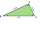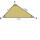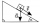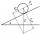# Similarity of triangles + angle - math problems

#### Number of problems found: 41

• SimilarityAre two right triangles similar to each other if the first one has an acute angle 70°, and the second one has an acute angle 20°?
• The trianglesThe triangles ABC and A'B'C 'are similar with a similarity coefficient of 2. The angles of the triangle ABC are alpha = 35°, beta = 48°. Determine the magnitudes of all angles of triangle A'B'C '.
• Similarity coefficientThe triangles ABC and A "B" C "are similar to the similarity coefficient 2. The sizes of the angles of the triangle ABC are α = 35° and β = 48°. Find the magnitudes of all angles of triangle A "B" C ".
• Two anglesThe triangles ABC and A'B'C 'are similar. In the ABC triangle, the two angles are 25° and 65°. Explain why in the triangle A'B'C 'is the sum of two angles of 90 degrees.
• ClimbRoad has climbing 1:27. How big is a angle corresponds to this climbing?
• Mast shadowMast has 13 m long shadow on a slope rising from the mast foot in the direction of the shadow angle at angle 15°. Determine the height of the mast, if the sun above the horizon is at angle 33°. Use the law of sines.
• Angle in RTDetermine the size of the smallest internal angle of a right triangle whose sides constitutes sizes consecutive members of arithmetic progressions.
• CosineCalculate the cosine of the smallest internal angle in a right-angled triangle with cathetus 3 and 8 and with the hypotenuse 8.544.
• Rectangular trapezoidThe ABCD rectangular trapezoid with the AB and CD bases is divided by the diagonal AC into two equilateral rectangular triangles. The length of the diagonal AC is 62cm. Calculate trapezium area in cm square and calculate how many differs perimeters of the
• MO Z7–I–6 2021In the triangle ABC, point D lies on the AC side and point E on the BC side. The sizes of the angles ABD, BAE, CAE and CBD are 30°, 60°, 20° and 30°, respectively. Find the size of the AED angle.
• Tower's viewFrom the church tower's view at the height of 65 m, the top of the house can be seen at a depth angle of alpha = 45° and its bottom at a depth angle of beta = 58°. Calculate the height of the house and its distance from the church.
• Area and two anglesCalculate the size of all sides and internal angles of a triangle ABC, if it is given by area S = 501.9; and two internal angles α = 15°28' and β = 45°.
• An observerAn observer standing west of the tower sees its top at an altitude angle of 45 degrees. After moving 50 meters to the south, he sees its top at an altitude angle of 30 degrees. How tall is the tower?
• A boyA boy of height 1.7m is standing 30m away from the flagstaff on the same level ground. He observes that the angle of deviation of the top of flagstaff is 30 degrees. Calculate the height of flagstaff.
• Inclined planeThe body stays on an inclined plane and exerts a compressive force of 70N on it. Find the angle between the inclined plane and the horizontal if a gravitational force of 100N acts on the body.
• Inclined planeOn the inclined plane with an inclination angle of 30°, we will put the body (fixed point) with mass 9 kg. Determine the acceleration of the body motion on an inclined plane.
• Diagonals at right angleIn the trapezoid ABCD, this is given: AB=12cm CD=4cm And diagonals crossed under a right angle. What is the area of this trapezoid ABCD?
• RailwaysRailways climb 7.4 ‰. Calculate the height difference between two points on the railway distant 3539 meters.
• RhombusABCD is a rhombus, ABD is an equilateral triangle and AC is equal to 4. Find the area of the rhombus.
• ClimbOn the road sign, which informs the climb is 8.7%. The car drive 5 km along this road. What is the height difference that the car went to?

Do you have an interesting mathematical word problem that you can't solve it? Submit a math problem, and we can try to solve it.

We will send a solution to your e-mail address. Solved examples are also published here. Please enter the e-mail correctly and check whether you don't have a full mailbox.

Please do not submit problems from current active competitions such as Mathematical Olympiad, correspondence seminars etc...
See also our trigonometric triangle calculator. Similarity of triangles - math problems. Angle Problems.